# JP2006180189A - Color processing method, color processing apparatus, and lookup table - Google Patents

## Info

Publication number
JP2006180189A
JP2006180189A JP2004371030A JP2004371030A JP2006180189A JP 2006180189 A JP2006180189 A JP 2006180189A JP 2004371030 A JP2004371030 A JP 2004371030A JP 2004371030 A JP2004371030 A JP 2004371030A JP 2006180189 A JP2006180189 A JP 2006180189A
Authority
JP
Japan
Prior art keywords
color
parameter
point representing
prediction model
color space
Prior art date
Legal status (The legal status is an assumption and is not a legal conclusion. Google has not performed a legal analysis and makes no representation as to the accuracy of the status listed.)
Withdrawn
Application number
JP2004371030A
Other languages
Japanese (ja)
Inventor
Makoto Sasaki

Original Assignee
Fuji Xerox Co Ltd

Priority date (The priority date is an assumption and is not a legal conclusion. Google has not performed a legal analysis and makes no representation as to the accuracy of the date listed.)
Filing date
Publication date
Application filed by Fuji Xerox Co Ltd, 富士ゼロックス株式会社 filed Critical Fuji Xerox Co Ltd
Priority to JP2004371030A priority Critical patent/JP2006180189A/en
Publication of JP2006180189A publication Critical patent/JP2006180189A/en
Application status is Withdrawn legal-status Critical

## Images

•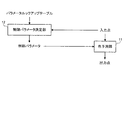•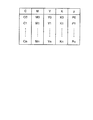•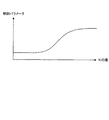•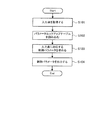•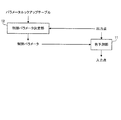••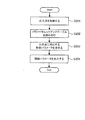•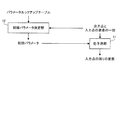••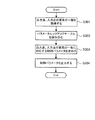•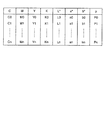•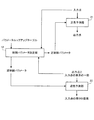•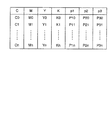## Abstract

PROBLEM TO BE SOLVED: To perform good color prediction without depending on a known characteristic.
A control parameter determining unit for determining a control parameter to be reflected in a color prediction model using a look-up table storing a control parameter for controlling the color prediction model, and the determined control parameter is reflected. The color in the color space (output color space) obtained by measuring the color reproduced by the device from the input point representing the color in the color space (input color space) for reproducing the color by the device using the color prediction model And a color predicting unit 11 that predicts an output point representing.
[Selection] Figure 1

## Description

The present invention relates to a color processing method for performing a process for predicting a color of a color reproduction device, and more particularly to a color processing method for predicting a color using a color prediction model.

In general, color reproduction devices such as a printer that reproduces color using four color components of CMYK and a display that reproduces color using three color components of RGB have different color reproduction characteristics depending on the characteristics of the device. . Therefore, for example, in a color copying machine that reads a color image with a scanner and prints it out in color, the RGB signal that is the output of the scanner is once converted into a CIE-L * a * b * or CIE that is a device-independent color space. Convert to -XYZ signal and convert it to CMYK signal which is input to printer. Therefore, in order to perform color reproduction satisfactorily, a combination of color components representing colors in the CMYK color space and RGB color space depending on the device and CIE-L * a * b * color independent of the device It is important to correctly associate combinations of color components representing colors in the space and the CIE-XYZ color space.

By the way, a color prediction model is used to perform such association. Usually, the color prediction model is based on a pair of color patches output by a color reproduction device and colorimetric values obtained by measuring the color patches with a colorimeter (hereinafter referred to as `` actual data pair ''). Color prediction is performed for colors that do not exist in the color patch.
Such color prediction models include, for example, a physical model such as the Neugebauer method and a model that learns actual data pairs using a neural network.
There has also been proposed a method for performing color prediction by performing regression analysis on real data pairs (see, for example, Patent Documents 1 and 2).

JP-A-10-262157 (page 4-8) JP 2002-84434 A (page 4-8, FIG. 1)

Among them, in the method of Patent Document 1, smooth weighting is applied to the actual data pair around the color to be predicted to enable prediction of a non-linear and smooth color as a whole. According to this method, prediction from CMYK (input color space) to L * a * b * (output color space), L * a * b * (output color space) and K (one of the point elements of the input color space) ) To CMY (the remaining point elements in the input color space) can be accurately performed. The same result can be obtained not only with a printer but also with an RGB system (display, scanner).
However, in this method, since the shape of the function representing the weighting coefficient in the weighted linear regression analysis is fixed, it is impossible to make an appropriate prediction in consideration of an area where the actual data is highly varied and the prediction becomes unstable.

Therefore, in Patent Literature 2, it is possible to control the smoothness of prediction for each color region. Generally, a printer has a property that prediction becomes unstable as K increases. For this reason, when L * a * b * and prediction from K to CMY are performed, it is difficult to ensure good accuracy with the same control when K is small and large. Therefore, Patent Document 2 proposes a prediction model having a parameter for controlling the smoothness of prediction according to K, and eliminates unstable prediction in a low lightness region (high K% region).

However, in an actual color reproduction device, it is not always possible to make an appropriate prediction by using such known characteristics (such as the characteristic that the prediction becomes unstable as K increases).
First, when the colorimeter is unstable, the actual data pairs themselves may vary greatly, and the characteristics of the actual data pairs may change from day to day depending on the state of the device and the temperature. Or, even in the same device, the characteristics of the actual data pair are generally different due to the individual difference.

In addition, among actual data pairs, there may be a mixture of patches with stable colorimetry and patches with unstable colorimetry. Therefore, when several patch sets are prepared, the density of patches may be different between the patch sets, and it may not be predicted well if the same parameters are used. There are cases where the actual data pairs around the color to be predicted are sparse and the colorimetric accuracy is poor, and the accuracy varies among the actual data pairs due to various factors.
For this reason, if color prediction is performed using a color prediction model having parameters formulated based on known characteristics as in Patent Document 2, there is a limit to the good color prediction. There is a problem.

The present invention has been made to solve the above technical problems, and an object of the present invention is to enable good color prediction without depending on known characteristics.
Another object of the present invention is to reflect an appropriate parameter for each color in the color prediction model.

For this purpose, in the present invention, parameters to be reflected in the color prediction model are stored in a lookup table and used. That is, the color processing method of the present invention is a method for predicting a point representing a color in a color space using a color prediction model, and represents a color using a look-up table storing parameters for controlling the color prediction model. Determining a specific parameter for predicting the point, and reflecting the specific parameter in the color prediction model to predict the point representing the color.

Here, the prediction of a point representing a color includes the following.
First, the color space (output color) obtained by measuring the color reproduced by a specific device from the input point representing the color in the color space (input color space) for reproducing the color by the specific device. The output point representing the color in the space is predicted.
Second, the color space (input color) for reproducing the color on the specific device from the output point representing the color in the color space (output color space) obtained by measuring the color reproduced on the specific device. The input point representing the color in the space is predicted.
Third, output points representing colors in the color space (output color space) obtained by measuring colors reproduced on a specific device, and the color space (input color) for reproducing colors on that specific device. The remaining elements of the input point are predicted from at least one of the elements of the input point representing the color in the space.

The present invention can also be understood as a device that performs color prediction by reflecting the parameters stored in the lookup table in the color prediction model. In this case, the color processing apparatus of the present invention is an apparatus that predicts a point representing a color in a color space, and stores a lookup table that stores parameters for controlling a color prediction model for predicting a point representing a color. A storage unit; a determination unit that determines a parameter for predicting a point representing a color using a lookup table stored in the storage unit; and a color prediction model that reflects the parameter determined by the determination unit Thus, a color prediction unit that predicts a point representing a color is provided.

Here, the prediction of a point representing a color includes the following.
First, the color space (output color) obtained by measuring the color reproduced by a specific device from the input point representing the color in the color space (input color space) for reproducing the color by the specific device. The output point representing the color in the space is predicted.
Second, the color space (input color) for reproducing the color on the specific device from the output point representing the color in the color space (output color space) obtained by measuring the color reproduced on the specific device. The input point representing the color in the space is predicted.
Third, output points representing colors in the color space (output color space) obtained by measuring colors reproduced on a specific device, and the color space (input color) for reproducing colors on that specific device. The remaining elements of the input point are predicted from at least one of the elements of the input point representing the color in the space.

On the other hand, the present invention can also be understood as a lookup table. In that case, the look-up table of the present invention has parameters for controlling a color prediction model for predicting a point representing a color in the color space, and the parameters are as follows.
First, it is stored for each input point representing a color in a color space (input color space) for reproducing a color by a specific device, and obtained by measuring the color reproduced by the specific device. This is a parameter for controlling a color prediction model when an output point representing a color in a color space (output color space) is predicted from the input point.
Second, it is stored for each output point representing a color in the color space (output color space) obtained by measuring the color reproduced by a specific device, and for reproducing the color on that specific device. This is a parameter for controlling a color prediction model when an input point representing a color in a color space (input color space) is predicted from its output point.
Third, output points representing colors in the color space (output color space) obtained by measuring colors reproduced on a specific device, and the color space (input color) for reproducing colors on that specific device. Is a parameter for controlling the color prediction model when predicting the remaining elements of the input point from the color information. .

According to the present invention, good color prediction can be performed without relying on known characteristics.

The best mode for carrying out the present invention (hereinafter referred to as “embodiment”) will be described below in detail with reference to the accompanying drawings. In the present embodiment, a color space for reproducing colors by a device is called an “input color space”, and a point representing a color in this space is called an “input point”. A space obtained by measuring colors reproduced by the device is called an “output color space”, and a point representing a color in this space is called an “output point”.

(First embodiment)
FIG. 1 is a diagram showing a functional configuration of the color processing apparatus according to the first embodiment of the present invention.
As shown in the figure, the color processing apparatus of the present embodiment includes a color prediction unit 11 and a control parameter determination unit 12.
The color prediction unit 11 is a part having a function of predicting an output point from an input point. The control parameter determination unit 12 is a part having a function of determining a control parameter to be reflected in the color prediction model according to an input point with reference to a parameter lookup table to be described later, and can also be grasped as a determination unit It is.
Each of these functions can be realized only by hardware, but can also be realized by a combination of hardware and software. In the latter case, each function is realized by a CPU (not shown) of the color processing apparatus reading and executing a program stored in a memory (not shown), for example. The parameter lookup table can also be realized by a memory (not shown).

Next, the parameter lookup table referred to by the control parameter determination unit 12 in the present embodiment will be described.
FIG. 2 shows an example of a parameter look-up table that stores control parameters that are reflected in a color prediction model that predicts output points in the L * a * b * color space from input points in the CMYK color space. In this parameter lookup table, CMYK grid points (Ci, Mi, Yi, Ki) are associated with control parameters Pi of the color prediction model corresponding to each grid point (i = 0, 1,..., N). ).
The parameter lookup table can be generated in advance by analyzing actual data of the color reproduction device. The actual data in this case is a pair of a CMYK patch and an L * a * b * value obtained by measuring this patch with a colorimeter. For example, the control parameter p for controlling the smoothness of prediction for sparse / dense is determined by examining the sparse / dense density of patches near the lattice points for all CMYK lattice points prepared for generating the parameter lookup table. can do.

Next, the control parameter p for controlling the smoothness of the prediction will be described in detail by showing a specific color prediction model.
Here, a color prediction model for predicting a three-dimensional color signal from the three-dimensional color signal disclosed in Patent Documents 1 and 2 will be considered. That is, it is assumed that real data (x 1i , x 2i , x 3i ) in the input color space and real data (y 1i , y 2i , y 3i ) in the output color space are obtained. The linear relationship between the data (x 1i , x 2i , x 3i ) and the predicted value (y ′ 1i , y ′ 2i , y ′ 3i ) for the actual data in the output color space includes a constant term as follows: It is assumed that they are tied together.

Thus, an arbitrary point (x 1j , x 2j , x 3j ) in the input color space and a predicted value (y ′ 1j , y ′ 2j , y ′ 3j ) for the point in the output color space are expressed by the following equations: It becomes such a relationship.

In this color prediction model, a weighted linear regression analysis is performed using real data for an arbitrary point (x 1j , x 2j , x 3j ) in the input color space. Specifically, the square sum of the weighted Euclidean distance between the actual data (y 1i , y 2i , y 3i ) and the predicted value (y ′ 1i , y ′ 2i , y ′ 3i ) as in the following equation: E j is obtained, and matrix components m 11 , m 12 ,... That minimize E j are obtained.

Here, W ij is defined as the following equation.

First, in the first equation, H is a composite function, and for example, operations such as sum and product can be used.
In the second and third expressions, F ij and G ij are monotonically decreasing functions. Therefore, W 1ij and W 2ij are set so that the influence is reduced by reducing the weight for real data having a large distance difference from the point of interest , while the real data having a small difference in distance from the point of interest is used. Is a function set to increase the influence by increasing the weight. Then, coefficients (hereinafter referred to as “weight widths”) for adjusting how much the difference in distance from the point of interest affects (x 10 , x 20 , x 30 ), (y 10 , y 20). , Y 30 ) to adjust the weight for the actual data.

By the way, in patent document 1, this weight width is fixed. On the other hand, in Patent Document 2, although this weight width is variable, for example, the weight width is obtained using a calculation formula determined by paying attention to characteristics such as prediction becoming unstable as K increases. ing.
On the other hand, in this embodiment, regardless of such characteristics, for example, when there is a region where the sparse density of patches near the lattice points is sparse, the weight is used to smooth the prediction. It is also possible to increase the width. That is, as shown in FIG. 3, the weight width (control parameter p) can be set to increase as the sparse density of the patches becomes sparse.
However, it should be noted here that FIG. 3 does not show the characteristic that, for example, the sparse density of the patch becomes sparse as the values of C, M, Y, and K increase. If the density is sparse, this indicates only a setting method of setting a large value as the control parameter p in the parameter lookup table.

As the control parameter p, any parameter in any color prediction model may be adopted. For example, a control parameter for causing the prediction result to follow actual data may be employed as a parameter that is contrary to the control parameter for smoothing the prediction.
Further, when determining the control parameter p, it may be determined by the intuition and experience of the designer, and this is a great advantage in the present embodiment.

Next, the operation of the color processing apparatus having such a functional configuration will be described in detail.
First, the operation of the control parameter determination unit 12 will be described.
FIG. 4 is a flowchart showing an example of the operation flow of the control parameter determination unit 12.
As shown in the figure, the control parameter determination unit 12 first acquires information on input points (step 101). Here, since the input point is represented by CMYK, values of C, M, Y, and K are acquired.
Then, the parameter lookup table is read (step 102).
Next, the control parameter determination unit 12 obtains a control parameter p for the combination of C, M, Y, and K values acquired in Step 101 (Step 103).
In this case, if a combination of C, M, Y, and K values is stored in the parameter lookup table, the control parameter p associated with the combination may be extracted. On the other hand, if the combination of C, M, Y, and K values is not stored in the parameter lookup table, the control parameter p is determined as follows.

Note that fCMYK → p represents a known lookup table interpolation function, but any function can be used as long as interpolation is possible. For example, local regression analysis can be performed using the correspondence between CMYK of the parameter lookup table and the control parameter p.
Finally, the acquired control parameter p is output to, for example, a storage device (not shown) (step 104).
After the control parameter p is determined in this manner, color prediction by the color prediction unit 11 is performed. The operation at this time is to acquire an input point in the CMYK color space and predict an output point in the L * a * b * color space from this input point as follows.

Here, F represents a color prediction model from the CMYK color space to the L * a * b * color space. This color prediction model reflects the control parameter p output in step 104. As the color prediction model, for example, those described in Patent Documents 1 and 2 can be used, but any other color prediction model may be used.

In the prior art, the control parameter p is constant for any input point or formulated for the input point. On the other hand, in the present embodiment, it is possible to perform color prediction with higher accuracy by making the control parameter p variable in this way.
Further, in the present embodiment, since the control parameter p can be held in the lookup table, there is an advantage that the parameter determination process is faster than the formulation and optimization each time prediction is performed.

(Second embodiment)
FIG. 5 is a diagram showing a functional configuration of the color processing apparatus according to the second embodiment of the present invention.
As shown in the figure, the color processing apparatus of the present embodiment includes a color prediction unit 11 and a control parameter determination unit 12. This embodiment is different from the first embodiment in which an output point is predicted from an input point in that an input point is predicted from an output point.
Each of these functions can be realized only by hardware, but can also be realized by a combination of hardware and software. In the latter case, each function is realized by a CPU (not shown) of the color processing apparatus reading and executing a program stored in a memory (not shown), for example. The parameter lookup table can also be realized by a memory (not shown).

Next, the parameter lookup table referred to by the control parameter determination unit 12 in the present embodiment will be described.
FIG. 6 shows an example of a parameter lookup table that stores control parameters that are reflected in a color prediction model that predicts input points in the RGB color space from output points in the L * a * b * color space. In this parameter lookup table, L * a * b * grid points (Li, ai, bi) are associated with control parameters Pi of the color prediction model corresponding to each grid point (i = 0, 1, ..., n). Here, L * a * b * in the parameter lookup table may be L * a * b * predicted using a color prediction model from RGB grid points.
Since the positioning of the control parameter p in the color prediction model is the same as that described in the first embodiment, detailed description thereof is omitted. The present embodiment can be applied to a display having RGB color components, for example.

Next, the operation of the color processing apparatus having such a functional configuration will be described in detail.
First, the operation of the control parameter determination unit 12 will be described.
FIG. 7 is a flowchart showing an example of the operation flow of the control parameter determination unit 12.
As shown in the figure, the control parameter determination unit 12 first acquires output point information (step 201). Here, since the output point is represented by L * a * b * , the values of L * , a * , and b * are acquired.
Then, the parameter lookup table is read (step 202).
Next, the control parameter determination unit 12 obtains the control parameter p for the combination of the values of L * , a * , and b * acquired in Step 201 (Step 203).
In this case, if the combination of the values of L * , a * , and b * is stored in the parameter lookup table, the control parameter p associated with the combination may be extracted. On the other hand, if the combination of the values of L * , a * , b * is not stored in the parameter lookup table, the control parameter p is determined as follows.

Note that f L * a * b * → p represents a known lookup table interpolation function, but any function can be used as long as interpolation is possible. Note that when L * a * b * predicted using a color prediction model from RGB grid points is used, the intervals are not equal as in the grid points, and the interpolation calculation is performed using L * a in the parameter lookup table. What is necessary is just to perform using a local regression analysis using the correspondence of * b * and the control parameter p, or a weighted average.
Finally, the acquired control parameter p is output to, for example, a storage device (not shown) (step 204).
After the control parameter p is determined in this manner, color prediction by the color prediction unit 11 is performed. The operation at this time is to obtain an output point in the L * a * b * color space and predict an input point in the RGB color space from this output point as follows.

Here, F represents a color prediction model from the RGB color space to the L * a * b * color space. However, in the present embodiment, color prediction is performed from the L * a * b * color space to the RGB color space, which is reverse prediction, and is represented by F- 1 . This color prediction model reflects the control parameter p output in step 104. As the color prediction model, for example, those described in Patent Documents 1 and 2 can be used, but any other color prediction model may be used.

In the prior art, the control parameter p is constant for any input point or formulated for the input point. On the other hand, in the present embodiment, it is possible to perform color prediction with higher accuracy by making the control parameter p variable in this way.
Further, in the present embodiment, since the control parameter p can be held in the lookup table, there is an advantage that the parameter determination process is faster than the formulation and optimization each time prediction is performed.

(Third embodiment)
FIG. 8 is a diagram showing a functional configuration of the color processing apparatus according to the third embodiment of the present invention.
As shown in the figure, the color processing apparatus of the present embodiment includes a color prediction unit 11 and a control parameter determination unit 12. For example, since the prediction from L * a * b * to CMYK is a prediction in the direction with many dimensions, in order to uniquely determine CMY, L * a * b * and K are given, and CMY is It is common to predict. For this reason, in this embodiment, the remaining elements of the input point are predicted from the output point and a part of the input point. This is different from the first embodiment in which the output point is predicted from the input point and the second embodiment in which the input point is predicted from the output point.
Each of these functions can be realized only by hardware, but can also be realized by a combination of hardware and software. In the latter case, each function is realized by a CPU (not shown) of the color processing apparatus reading and executing a program stored in a memory (not shown), for example. The parameter lookup table can also be realized by a memory (not shown).

Next, the parameter lookup table referred to by the control parameter determination unit 12 in the present embodiment will be described.
FIG. 9 shows control parameters reflected in a color prediction model that predicts CMY as the remaining elements of the input point from the output point in the L * a * b * color space and K as a part of the element of the input point. Is an example of a parameter look-up table that stores. In this parameter lookup table, combinations of L * a * b * lattice points and K (Li, ai, bi, Ki) are associated with control parameters Pi of the color prediction model corresponding to each combination (i = 0, 1, ..., n). Here, L * a * b * in the parameter lookup table may be L * a * b * predicted using a color prediction model from RGB grid points.
Since the positioning of the control parameter p in the color prediction model is the same as that described in the first embodiment, detailed description thereof is omitted.

Next, the operation of the color processing apparatus having such a functional configuration will be described in detail.
First, the operation of the control parameter determination unit 12 will be described.
FIG. 10 is a flowchart illustrating an example of the operation flow of the control parameter determination unit 12.
As shown in the figure, the control parameter determination unit 12 first obtains output point information and information on a part of elements of the input point (step 301). Here, since the output point is represented by L * a * b * and some of the elements of the input point are represented by K, the values of L * , a * , b * , and K are acquired.
Then, the parameter lookup table is read (step 302).
Next, the control parameter determination unit 12 obtains a control parameter p for the combination of L * , a * , b * , and K values acquired in Step 301 (Step 303).
In this case, if a combination of L * , a * , b * , and K values is stored in the parameter lookup table, the control parameter p associated with the combination may be extracted. On the other hand, if the combination of the values of L * , a * , b * , and K is not stored in the parameter lookup table, the control parameter p is determined as follows.

Note that f L * a * b * K → p represents a known function for performing lookup table interpolation, but any function can be used as long as interpolation can be performed. In the case of using the L * a * b * were predicted using color prediction model from RGB grid points, because there is no more evenly spaced as grid points, interpolation calculation, parameter lookup tables L * a It may be performed using a local regression analysis using a correspondence between * b * and the control parameter p, or a weighted average.
Finally, the acquired control parameter p is output to a storage device (not shown), for example (step 304).
After the control parameter p is determined in this way, the color prediction unit 11 performs color prediction. The operation at this time is to obtain K as part of the elements of the output point and the input point in the L * a * b * color space and predict the rest of the elements of the input point from this output point as follows. Is.

Here, F represents a color prediction model from the CMY color space to the L * a * b * color space and K. However, in the present embodiment, color prediction from the L * a * b * color space and K to the CMY color space, which is an inverse prediction, is represented by F- 1 . This color prediction model reflects the control parameter p output in step 104. As the color prediction model, for example, those described in Patent Documents 1 and 2 can be used, but any other color prediction model may be used.

By the way, in this embodiment, a parameter lookup table (hereinafter referred to as “LabKp table”) in which a combination of L * a * b * lattice points and K is associated with a control parameter p is provided, and L * , a The control parameter p is obtained from the values of * , b * and K. However, when the value of K can be calculated using a function for obtaining the value of K from the L * a * b * value, for example, a UCR (Under Color Removal) function, it is not always necessary to use such a parameter lookup table. Absent. That is, in such a case, a parameter lookup table (hereinafter referred to as “Labp table”) in which L * a * b * lattice points are associated with the control parameter p, or a value of K and the control parameter p An associated parameter lookup table (hereinafter referred to as “Kp table”) may be provided, and the control parameter p may be obtained from either the L * a * b * value or the K value. Alternatively, selection means may be provided for selecting whether to use the LabKp table, the Labp table, or the Kp table according to the determination result as to whether or not the device calculates the value of K using the UCR function or the like.

Further, the parameter lookup table in the present embodiment may be a lookup table in which CMYK values, L * a * b * values, and control parameters p are associated with each other as shown in FIG. Specifically, if CMYK is a grid point, L * a * b * is a value calculated by the color prediction model, and the parameter used when calculating L * a * b * is stored as the control parameter p. Good. In Patent Documents 1 and 2, when performing inverse prediction such as obtaining CMY from L * a * b * and K, there is a process of sequentially calculating CMY several times. Finally, a method of converging to CMY that minimizes an error from actual data is adopted. In such a case, if the parameter lookup table as shown in FIG. 11 is provided, the control parameter p can be determined by [Equation 9] for the first CMY of the sequential processing. The control parameter p can be determined using Equation 5], and the accuracy of sequential processing is also improved.

In the prior art, the control parameter p is constant for any input point or formulated for the input point. On the other hand, in the present embodiment, it is possible to perform color prediction with higher accuracy by making the control parameter p variable in this way.
Further, in the present embodiment, since the control parameter p can be held in the lookup table, there is an advantage that the parameter determination process is faster than the formulation and optimization each time prediction is performed.

(Fourth embodiment)
FIG. 12 is a diagram showing a functional configuration of the color processing apparatus according to the fourth embodiment of the present invention.
As shown in the figure, the color processing apparatus according to the present embodiment includes a control parameter determination unit 12, a normal color prediction unit 21, and a reverse color prediction unit 22.
The control parameter determination unit 12 refers to the parameter look-up table, determines a positive control parameter used for the color prediction model in the normal color prediction unit 21 according to the input point, and sets a part of the elements of the output point and the input point. Accordingly, it is a part having a function of determining an inverse control parameter used in the color prediction model in the inverse color prediction unit 22 and can be grasped as a determination unit. Further, the normal color predicting unit 21 has a function of predicting an output point from an input point, and the reverse color predicting unit 22 has a function of predicting the remaining elements of the input point from a part of the elements of the output point and the input point. These are parts that can be grasped as the color prediction part.
Each of these functions can be realized only by hardware, but can also be realized by a combination of hardware and software. In the latter case, each function is realized by a CPU (not shown) of the color processing apparatus reading and executing a program stored in a memory (not shown), for example. The parameter lookup table can also be realized by a memory (not shown).

Next, the parameter lookup table referred to by the control parameter determination unit 12 in the present embodiment will be described.
In this embodiment, the input color space is CMYK and the output color space is L * a * b * . The normal color prediction unit 21 uses a color prediction model that predicts L * a * b * from CMYK, and the reverse color prediction unit 22 predicts CMY from L * a * b * and K. Shall be used. However, the present embodiment is not limited to this, and any model may be used as long as it is a similar color prediction system.
Here, if the parameter look-up table is as shown in FIG. 11, the control parameters of the color prediction model for normal color prediction and reverse color prediction can be determined by one look-up table.

Next, the operation of the color processing apparatus having such a functional configuration will be described in detail.
First, the control parameter determination unit 12 performs the same operation as described in the first embodiment, and outputs a positive control parameter. Then, the normal color prediction unit 21 acquires an input point in the CMYK color space, and predicts an output point in the L * a * b * color space from this input point.
Thereafter, the control parameter determination unit 12 performs the same operation as described in the third embodiment, and outputs a reverse control parameter. At this time, the control parameter determination unit 12 is supplied with the L * a * b * value of the output point predicted by the normal color prediction unit 21 and a part of the elements of the input point input to the normal color prediction unit 21. A certain K is input. The reverse color prediction unit 22 also acquires these pieces of information and predicts the remaining elements of the input points.

The reversible accuracy of the inverse color prediction model F- 1 with respect to the normal color prediction model F can also be evaluated by the configuration shown in FIG. In this case, when CMY is calculated by the reverse color prediction unit 22 using L * a * b * output from the normal color prediction unit 21 and K input to the normal color prediction unit 21, the normal color prediction unit The color prediction model may be evaluated based on the distance from CMY input to 21.
When calculating a parameter with good reversibility using such a configuration, the control parameter p for each CMYK lattice point in the parameter lookup table may be set in the following range, for example.

That is, using the parameters in such a range, L * a * b * is predicted using [Expression 6] from the grid point CMYK, and [Expression 9] is calculated from the predicted L * a * b * and K. CMY can be predicted by searching for a parameter that minimizes the distance between the grid point CMY and the predicted CMY. Then, the accuracy of the color prediction model is guaranteed by storing the CMYK lattice points at this time, the predicted L * a * b *, and the control parameter p in the parameter lookup table of FIG. A parameter lookup table can be generated.

In the first to fourth embodiments described above, the control parameters stored in the parameter lookup table are described as scalars. However, as shown in FIG. 13, the control parameter may be a vector. FIG. 13 shows an example in which three control parameters are provided for CMYK lattice points. In this case, the control parameter p is determined as follows.

Note that f CMYK → p (for convenience of description, the vector p is simply “p”) indicates a known lookup table interpolation function, but any function can be used as long as interpolation is possible. This interpolation calculation can also be realized by a known method such as weighted average or local regression analysis.
By the way, Patent Document 2 also gives an example in which the weight widths in the second and third equations of [Equation 4] are set for the C, M, and Y axes. The vector-type control parameters shown in FIG. 13 can be applied to such C, M, and Y axes.

In the present embodiment, the hardware for performing color processing is not particularly limited, but may be realized by a computer such as a personal computer, or may be realized by an image processing unit (IPS) of the image forming apparatus. ) May be realized.

It is the block diagram which showed the function structure of the 1st Embodiment of this invention. It is the figure which showed an example of the parameter look-up table referred in the 1st Embodiment of this invention. It is a figure for demonstrating the setting of the control parameter with respect to the parameter lookup table referred in the 1st thru | or 4th Embodiment of this invention. It is the flowchart which showed the operation | movement of the control parameter determination part in the 1st Embodiment of this invention. It is the block diagram which showed the function structure of the 2nd Embodiment of this invention. It is the figure which showed an example of the parameter look-up table referred in the 2nd Embodiment of this invention. It is the flowchart which showed the operation | movement of the control parameter determination part in the 2nd Embodiment of this invention. It is the block diagram which showed the function structure of the 3rd Embodiment of this invention. It is the figure which showed an example of the parameter look-up table referred in the 3rd Embodiment of this invention. It is the flowchart which showed the operation | movement of the control parameter determination part in the 3rd Embodiment of this invention. It is the figure which showed an example of the parameter look-up table referred by the 4th Embodiment etc. of this invention. It is the block diagram which showed the function structure of the 4th Embodiment of this invention. It is the figure which showed an example of the parameter lookup table which can be referred in the 1st thru | or 4th Embodiment of this invention.

Explanation of symbols

DESCRIPTION OF SYMBOLS 11 ... Color prediction part, 12 ... Control parameter determination part, 21 ... Normal color prediction part, 22 ... Reverse color prediction part

## Claims (13)

1. In a color processing method for predicting a point representing a color in a color space using a color prediction model,
Determining specific parameters for predicting the point representing the color using a look-up table storing parameters controlling the color prediction model;
Reflecting the specific parameter in the color prediction model and predicting a point representing the color.
2. The step of predicting comprises:
Obtaining an input point representing a color in a color space for reproducing the color on a specific device;
The color processing method according to claim 1, further comprising: predicting an output point representing a color in a color space obtained by measuring a color reproduced by the specific device from the input point. .
3. The step of predicting comprises:
Obtaining an output point representing a color in a color space obtained by measuring a color reproduced by a specific device;
The color processing method according to claim 1, further comprising: predicting an input point representing a color in a color space for reproducing a color by the specific device from the output point.
4. The step of predicting comprises:
An output point representing a color in a color space obtained by measuring colors reproduced by a specific device, and at least one element of an input point representing a color in a color space for reproducing the color by the specific device; Obtaining color information consisting of:
The color processing method according to claim 1, further comprising: predicting the remaining elements of the input point from the color information.
5.   The color processing method according to claim 1, wherein the parameter is a parameter for controlling smoothness of prediction by the color prediction model or followability of a prediction result with respect to actual data that is a basis of prediction.
6. In a color processing apparatus that predicts points representing colors in a color space,
A storage unit that stores a lookup table that stores parameters for controlling a color prediction model for predicting the point representing the color;
A determination unit that determines a parameter for predicting a point representing the color using the lookup table stored in the storage unit;
A color processing apparatus comprising: a color prediction unit that predicts a point representing the color by a color prediction model reflecting the parameter determined by the determination unit.
7. The color prediction unit
Obtain an input point representing the color in the color space for reproducing the color on a specific device,
The color processing apparatus according to claim 6, wherein an output point representing a color in a color space obtained by measuring a color reproduced by the specific apparatus is predicted from the input point.
8. The color prediction unit
Obtain an output point that represents the color in the color space obtained by measuring the color reproduced by a specific device,
The color processing apparatus according to claim 6, wherein an input point representing a color in a color space for reproducing a color by the specific apparatus is predicted from the output point.
9. The color prediction unit
An output point representing a color in a color space obtained by measuring colors reproduced by a specific device, and at least one element of an input point representing a color in a color space for reproducing the color by the specific device; Get color information consisting of
The color processing apparatus according to claim 6, wherein the remaining elements of the input point are predicted from the color information.
10.   The color processing apparatus according to claim 6, wherein the parameter is a parameter that controls smoothness of prediction by the color prediction model or followability of a prediction result with respect to actual data that is a basis of prediction.
11. Parameters controlling a color prediction model for predicting points representing colors in a color space;
The parameter is
Stored for each input point representing a color in a color space for color reproduction on a particular device,
Lookup characterized by being a parameter for controlling the color prediction model when an output point representing a color in a color space obtained by measuring colors reproduced by the specific device is predicted from the input point table.
12. Parameters controlling a color prediction model for predicting points representing colors in a color space;
The parameter is
Stored for each output point representing a color in a color space obtained by measuring the color reproduced by a specific device,
A lookup table, which is a parameter for controlling the color prediction model when an input point representing a color in a color space for reproducing a color by the specific device is predicted from the output point.
13. Parameters controlling a color prediction model for predicting points representing colors in a color space;
The parameter is
An output point representing a color in a color space obtained by measuring colors reproduced by a specific device, and at least one element of an input point representing a color in a color space for reproducing the color by the specific device; Is stored for each color information consisting of
The look-up table, which is a parameter for controlling the color prediction model when the remaining elements of the input point are predicted from the color information.
JP2004371030A 2004-12-22 2004-12-22 Color processing method, color processing apparatus, and lookup table Withdrawn JP2006180189A (en)

## Priority Applications (1)

Application Number Priority Date Filing Date Title
JP2004371030A JP2006180189A (en) 2004-12-22 2004-12-22 Color processing method, color processing apparatus, and lookup table

## Applications Claiming Priority (1)

Application Number Priority Date Filing Date Title
JP2004371030A JP2006180189A (en) 2004-12-22 2004-12-22 Color processing method, color processing apparatus, and lookup table

## Publications (1)

Publication Number Publication Date
JP2006180189A true JP2006180189A (en) 2006-07-06

# Family

## Family Applications (1)

Application Number Title Priority Date Filing Date
JP2004371030A Withdrawn JP2006180189A (en) 2004-12-22 2004-12-22 Color processing method, color processing apparatus, and lookup table

## Country Status (1)

JP (1) JP2006180189A (en)

## Cited By (1)

* Cited by examiner, † Cited by third party
Publication number Priority date Publication date Assignee Title
JP2009296140A (en) * 2008-06-03 2009-12-17 Ricoh Co Ltd Image processor, program, and image processing method

## Cited By (1)

* Cited by examiner, † Cited by third party
Publication number Priority date Publication date Assignee Title
JP2009296140A (en) * 2008-06-03 2009-12-17 Ricoh Co Ltd Image processor, program, and image processing method

## Similar Documents

Publication Publication Date Title
US7035454B2 (en) Image processing method and apparatus, and profile management method
EP0637731B1 (en) Method of color reproduction
US8243325B2 (en) Method for prepress-time color match verification and correction
JP4590424B2 (en) Color processing apparatus and method
US8023156B2 (en) Image output color management system and method
US5543940A (en) Method and apparatus for converting color scanner signals into colorimetric values
JP4009812B2 (en) Image processing apparatus, computer-readable storage medium, and image processing method
US7583405B2 (en) Method for remote proofing of DFE color architecture
US7990592B2 (en) Methods and systems to produce consistent spot colors for multi-color print engines
US8842335B2 (en) Apparatus, method, and non-transitory computer readable medium to achieve appropriate color reproduction characteristics
US8379267B2 (en) Method to retrieve a gamut mapping strategy
US7692831B2 (en) Image processing apparatus and method thereof
CN103139443B (en) Color processing apparatus, color processing method and configuration file generation method
US6947589B2 (en) Dynamic gamut mapping selection
DE10311712B4 (en) Color processing method, color processing device, storage medium, color processing program and image forming device
US8477395B2 (en) Computation of gamut boundary by sampling device-independent color space
JP4595734B2 (en) Profile creation method, profile creation device, profile creation program, print control method, print control device, and print control program
JP4757078B2 (en) Color processing method and apparatus
US5539522A (en) Color copying machine provided with color correcting circuit
JP2004005566A (en) Color compiling apparatus and color compiling method
US7199900B2 (en) Color conversion coefficient preparation apparatus, color conversion coefficient preparation method, storage medium, and color conversion system
JP3417051B2 (en) Color information processing method and apparatus using feature parameter values independent of light source
US20050264837A1 (en) Color conversion method
JP2006217150A (en) Smoothing of lattice point arrangement
US20020168104A1 (en) Profile correction apparatus and profile correction program storage medium

## Legal Events

Date Code Title Description
A621 Written request for application examination

Free format text: JAPANESE INTERMEDIATE CODE: A621

Effective date: 20071120

A977 Report on retrieval

Free format text: JAPANESE INTERMEDIATE CODE: A971007

Effective date: 20091007

A131 Notification of reasons for refusal

Free format text: JAPANESE INTERMEDIATE CODE: A131

Effective date: 20091027

A521 Written amendment

Free format text: JAPANESE INTERMEDIATE CODE: A523

Effective date: 20091218

A761 Written withdrawal of application

Free format text: JAPANESE INTERMEDIATE CODE: A761

Effective date: 20100225